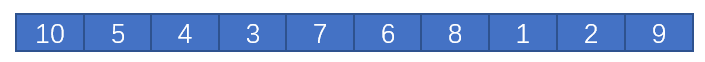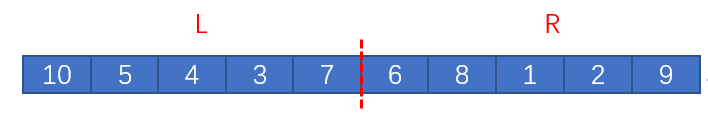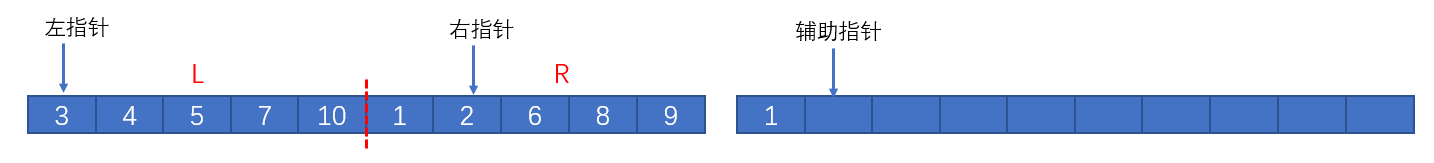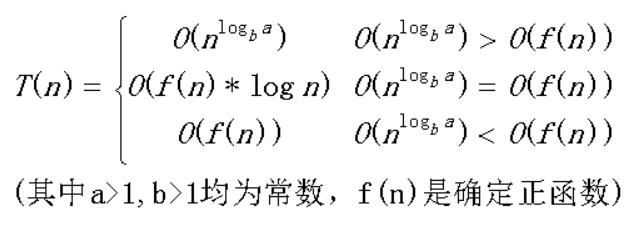# 都2020年了，听说你还不会归并排序？手把手教你手写归并排序算法

#### 递归方法的一般写法

1. 明确递归方法的功能边界；
2. 得到递归的递推关系；
3. 给定递归的终止条件。

#### 手写归并排序

private static void mergeSort(int[] arr,int left,int right)；[外链图片转存失败,源站可能有防盗链机制,建议将图片保存下来直接上传(img-vGvurbXm-1579178474078)(http://qiniu.exzlc.xyz/blog/sort-3.png)]/**
*
* @param arr 要合并的数组
* @param left 左边界
* @param mid 中间的分界
* @param right 右边界
*/
private static void merge(int[] arr,int left,int mid,int right){
int[] helpArr = new int[right - left + 1];//首先定义一个辅助数组
int lPoint = left;//左指针
int rPoint = mid  + 1;//右指针
int i = 0;//辅助指针
while(lPoint <= mid && rPoint <= right){//比较并填充辅助数组
if(arr[lPoint] <=  arr[rPoint])
helpArr[i++] =  arr[lPoint++];
else
helpArr[i++] =  arr[rPoint++];
}
while(lPoint <= mid){//将剩余元素填充至辅助数组
helpArr[i++] =  arr[lPoint++];
}
while(rPoint <= right){
helpArr[i++] =  arr[rPoint++];
}
for(int j = 0;j < helpArr.length;j ++){//将辅助数组中的元素回填至原数组
arr[left + j] = helpArr[j];
}
}


private static void mergeSort(int[] arr,int left,int right){
if(arr == null || right == left)//终止条件
return ;
int mid = left + (right - left) / 2;//确定分割的边界
mergeSort(arr,left,mid);//对左半部分调用递归方法，使其有序
mergeSort(arr,mid + 1,right);//对右半部分调用递归方法，使其有序
merge(arr,left,mid,right);//合并左右两部分，使整个数组有序
}


	/**
* 归并排序算法
* @param arr
*/
public static void mergeSort(int[] arr){
mergeSort(arr,0,arr.length - 1);//调用写好的递归版归并排序方法
}


#### 性能分析©️2019 CSDN 皮肤主题: 书香水墨 设计师: CSDN官方博客